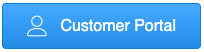Call or Text Us 24/7 941-485-2222If you're trying to decide whether to invest in a more energy-efficient appliance or you'd like to determine your electricity loads, you may want to estimate appliance energy consumption.

#### FORMULA FOR ESTIMATING ENERGY CONSUMPTION

Use this formula to estimate an appliance's energy use:

(Wattage × Hours Used Per Day) ÷ 1000 = Daily Kilowatt-hour (kWh) consumption

1 kilowatt (kW) = 1,000 Watts

Multiply this by the number of days you use the appliance during the year for the annual consumption in kWh per year.

#### ESTIMATING ANNUAL COST TO RUN AN APPLIANCE

Multiply the annual consumption in kWh per year (that you calculated above) by your local utility's rate per kWh consumed to calculate the annual cost to run an appliance. Note: To estimate the number of hours that a refrigerator actually operates at its maximum wattage, divide the total time the refrigerator is plugged in by three. Refrigerators, although turned "on" all the time, actually cycle on and off as needed to maintain interior temperatures.

##### EXAMPLES:

Window fan:
(200 Watts × 4 hours/day × 120 days/year) ÷ 1000
= 96 kWh × 11 cents/kWh
= \$10.56/year

Personal Computer and Monitor:
[(120 Watts + 150 Watts) × 4 hours/day × 365 days/year] ÷ 1000
= 394 kWh × 11 cents/kWh
= \$43.34/year

#### WATTAGE

You can usually find the wattage of most appliances stamped on the bottom or back of the appliance, or on its nameplate. The wattage listed is the maximum power drawn by the appliance. Since many appliances have a range of settings (for example, the volume on a radio), the actual amount of power consumed depends on the setting used at any one time.

If the wattage is not listed on the appliance, you can still estimate it by finding the current draw (in amperes) and multiplying that by the voltage used by the appliance. Most appliances in the United States use 120 volts. Larger appliances, such as clothes dryers and electric cooktops, use 240 volts. The amperes might be stamped on the unit in place of the wattage. If not, find a clamp-on ammeter -- an electrician's tool that clamps around one of the two wires on the appliance -- to measure the current flowing through it. You can obtain this type of ammeter in stores that sell electrical and electronic equipment. Take a reading while the device is running; this is the actual amount of current being used at that instant.

When measuring the current drawn by a motor, note that the meter will show about three times more current in the first second that the motor starts than when it is running smoothly.

Many appliances continue to draw a small amount of stand-by power when they are switched "off." These "phantom loads" occur in most appliances that use electricity, such as VCRs, televisions, stereos, computers, and kitchen appliances. Most phantom loads will increase the appliance's energy consumption a few watt-hours. These loads can be avoided by unplugging the appliance or using a power strip and using the switch on the power strip to cut all power to the appliance.

#### TYPICAL WATTAGES OF VARIOUS APPLIANCES

Here are some examples of the range of nameplate wattages for various household appliances:

• Aquarium = 50–1210 Watts
• Coffee maker = 900–1200
• Clothes washer = 350–500
• Clothes dryer = 1800–5000
• Dishwasher = 1200–2400 (using the drying feature greatly increases energy consumption)
• Dehumidifier = 785
• Electric blanket (Single/Double) = 60 / 100
• Fans
Ceiling = 65–175
Window = 55–250
Furnace = 750
Whole house = 240–750
• Hair dryer = 1200–1875
• Heater (portable) = 750–1500
• Clothes iron = 1000–1800
• Microwave oven = 750–1100
• Personal computer
CPU - awake / asleep = 120 / 30 or less
Monitor - awake / asleep = 150 / 30 or less
Laptop = 50
• Refrigerator (frost-free, 16 cubic feet) = 725
• Televisions (color)
• 19" = 65–110
• 27" = 113
• 36" = 133
• 53" - 61" Projection = 170
• Flat screen = 120
• Toaster = 800–1400
• Toaster oven = 1225
• VCR/DVD = 17–21 / 20–25
• Vacuum cleaner = 1000–1440
• Water heater (40 gallon) = 4500–5500
• Water pump (deep well) = 250–1100
• Water bed (with heater, no cover) = 120–380